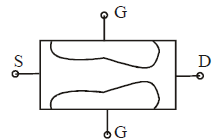# Test: BJT & FET

## 25 Questions MCQ Test Basic Electronics Engineering for SSC JE (Technical) | Test: BJT & FET

Description
Attempt Test: BJT & FET | 25 questions in 50 minutes | Mock test for Electrical Engineering (EE) preparation | Free important questions MCQ to study Basic Electronics Engineering for SSC JE (Technical) for Electrical Engineering (EE) Exam | Download free PDF with solutions
QUESTION: 1

Solution:
QUESTION: 2

Solution:
QUESTION: 3

### The collector and emitter currents levels for atransistor with common base dc current gain of 0.99 and base current of 20mA are respectively.

Solution:
QUESTION: 4

The CE amplifier circuit are preferred over CB amplifier circuit because they have

Solution:
QUESTION: 5

5. I npn - transistors are preferred over pnptransistors?

Solution:
QUESTION: 6

μF & μI denotes the forward and Inverted mode current gains of a BJT, which one of the following is correct ?

Solution:
QUESTION: 7

The Early effect in a bipolar junction transistor iscaused by

Solution:
QUESTION: 8

The phenomenon known as "Early effect" in abipolar transistor refers to a reduction of theeffective base-width caused by

Solution:
QUESTION: 9

The breakdown voltage of a transistor with its base open is BVCBOthen

Solution:
QUESTION: 10

For a BJT, the common base current gain a =0.98 and the collector base junction reverse biassaturation current ICO = 0.6mA. This BJT isconnected in the common emitter mode andoperated in the active region with a base drivecurrent IB = 20mA. The collector current IC forthis mode of operation is

Solution:
QUESTION: 11

The action of a JFET in its equivalent circuit canbest be represented as a

Solution:
QUESTION: 12

In a biased JFET, the shape of the channel is as shown in the given figure becauseSolution:
QUESTION: 13

Consider the following statements:
FETs when compared to BJTs have
1. High input resistance
2. Current follow due to majority carries
3. Low input impedance
4. Current flow due to minority carries

Q.

Which of the statements given above correct?

Solution:
QUESTION: 14

Compared to the bipolar junction transistor, a JFET;
1. Has a larger gain bandwidth production
2. Is less noisy
3. Has less input resistance
4. Has current flow due to only majority carriers

Solution:
QUESTION: 15

A FET is a better chopper than a BJT because ithas

Solution:
QUESTION: 16

The drain - source voltage at which drain currentbecomes nearly constant is called

Solution:
QUESTION: 17

The pinch off voltage VP = – 6V for P – channel JFET. If VGS = + 2V, what is the value of VDS at which it will enter into saturation region ?

Solution:
QUESTION: 18

An n = channel JFET has IDSS = 2mA, Vp = – 4V; Its transconductance gm (in mA/V) for an applied gate to source voltage VGS of – 2V is

Solution:
QUESTION: 19

An N- channel JFET, having a pinch – off voltage (Vp) of – 5V shows a transconductance (gm) of 1mA/V. When the applied gate to source voltage (VGS) is – 1V. Its maximum transconductance(in mA/V) is

Solution:
QUESTION: 20

The data sheet for a certain JFET (Junction Field Effect Transistor) indicates that IDSS (drain to source current with gate shorted) = 15 mA and VGS (off) (Cut – off value of gate to source voltage) = – 5V.

Solution:
QUESTION: 21

JFET has main drawback of

Solution:
QUESTION: 22

Thermal runaway is not encountered product

Solution:
QUESTION: 23

A junction FET, can be used as a voltage variableresistor.

Solution:
QUESTION: 24

When transistors are used in digital circuits they usually operate in the:

Solution:
QUESTION: 25

The input/output relationship of the common-collector and common-base amplifiers is:

Solution:Use Code STAYHOME200 and get INR 200 additional OFF Use Coupon Code